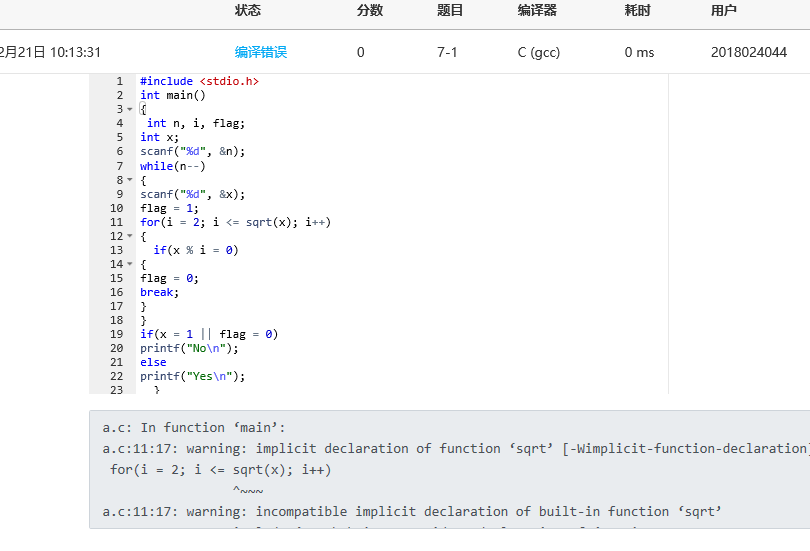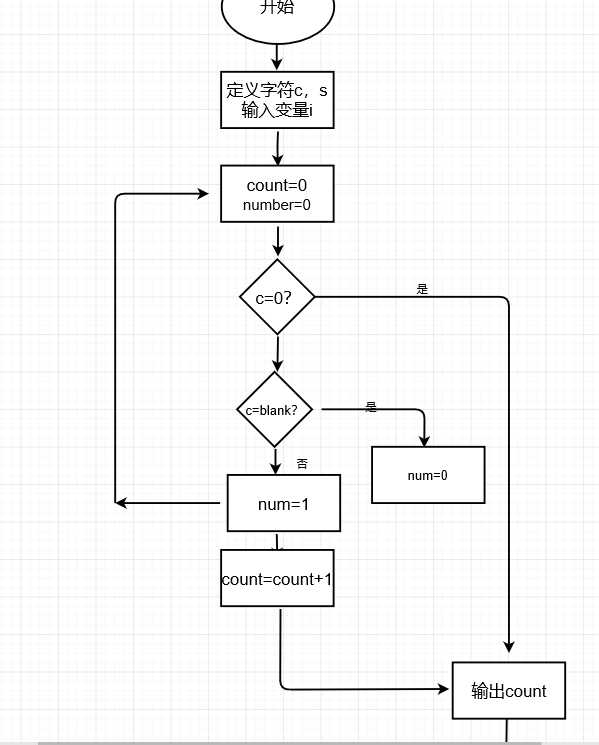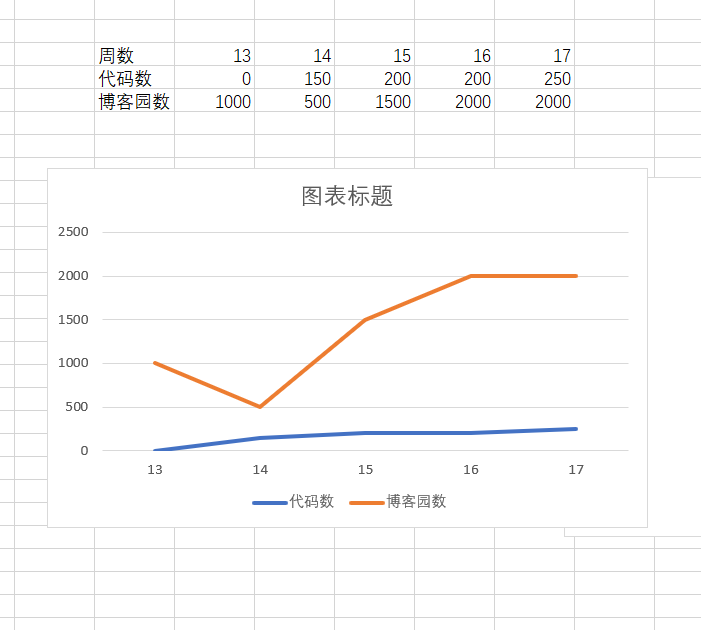7.1判断素数

1，代码如下

#include<stdio.h>
#include<math.h>
int main()
{
int i, N, a, b;
scanf("%d", &N);
for(b=1; b<=N; b++){
scanf("%d", &a);
for(i=2; i<=sqrt(a); i++)
if(a% i == 0)
break;
if(i>sqrt(a) && a !=1)
printf("Yes\n");
else
printf("No\n");
}
return 0;
}

2，设计思路；

（1）首先定义i,n,a,b,

(2)定义b，控制for循环，输入n个数

（3）判断是否为素数

（4）输出yes或no，结束循环

3，流程图；(3)本题调试过程遇到的问题及解决办法在编程过程中，对=表示赋值，==表示等于忽略了其中的问题，导致程序错误，修改后即可。

7-2 统计一行文本的单词个数

1，代码如下

#include<stdio.h>
int main(){
char c,s;
int i,count=0,number=0;
gets(s);
for(i=0;;i++)
{
c = s[i];
if(c=='\0')
break;
if(c==' ')number=0;
else if(number == 0)
{
number = 1;
count++;
}
}
printf("%d",count);
return 0;
}

2，思路如下；

（1）首先定义c，s

（2）运用for循环，定义变量i的量

（3）利用多个循环，count计数

（4）输出结果，结束

3，流程图；(3)本题调试过程遇到的问题及解决办法；无

1、总结这几周所学内容

2，点评同学的作业；点评于海龙作业点评肖晨东的作业点评刘铭的作业posted on 2018-12-22 18:59  贾振伟  阅读(228)  评论(6编辑  收藏  举报